# Circles, sectors, rings, buffers, and the n-gons

1060
0
01-04-2017 07:07 AM
Labels (1)byMVP Legendary Contributor
1 0 1,060

Code posted on my GitHub repository... called circle_make.py, perhaps a bit of a misnomer since it works to create all things associated with 'circular' features, which would include ellipses, triangles, squares, rectangles, sectors, arcs, pentagons, hexagons, octagons and n-gons... anything whose points can be placed on a circle.

Here are some pictures, you can examine the code at your leisure.  The functions (def) can be used in scripts to work with arcpy, numpy and with some stretching... the field calculator.  If you have any useful examples, pass them on.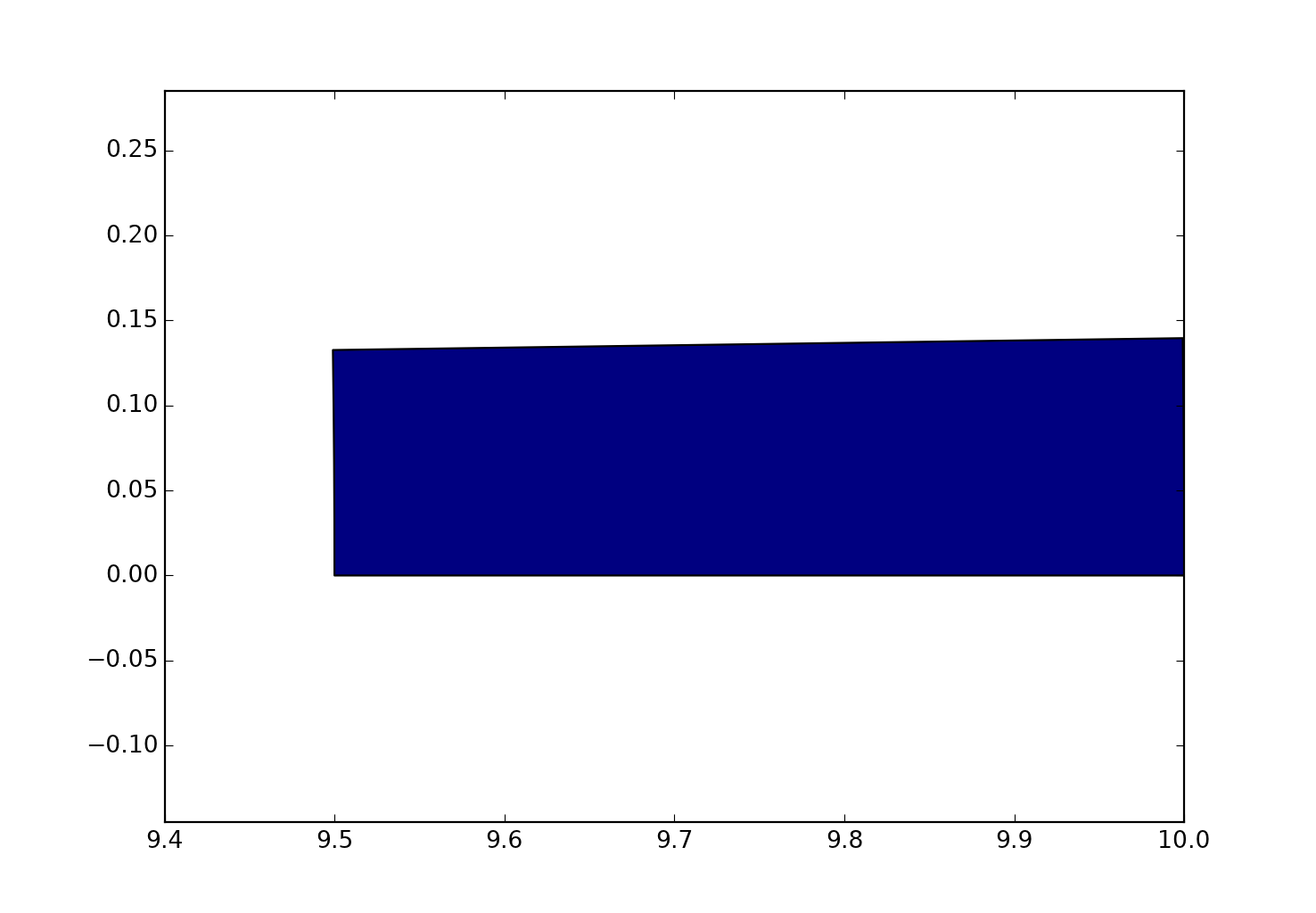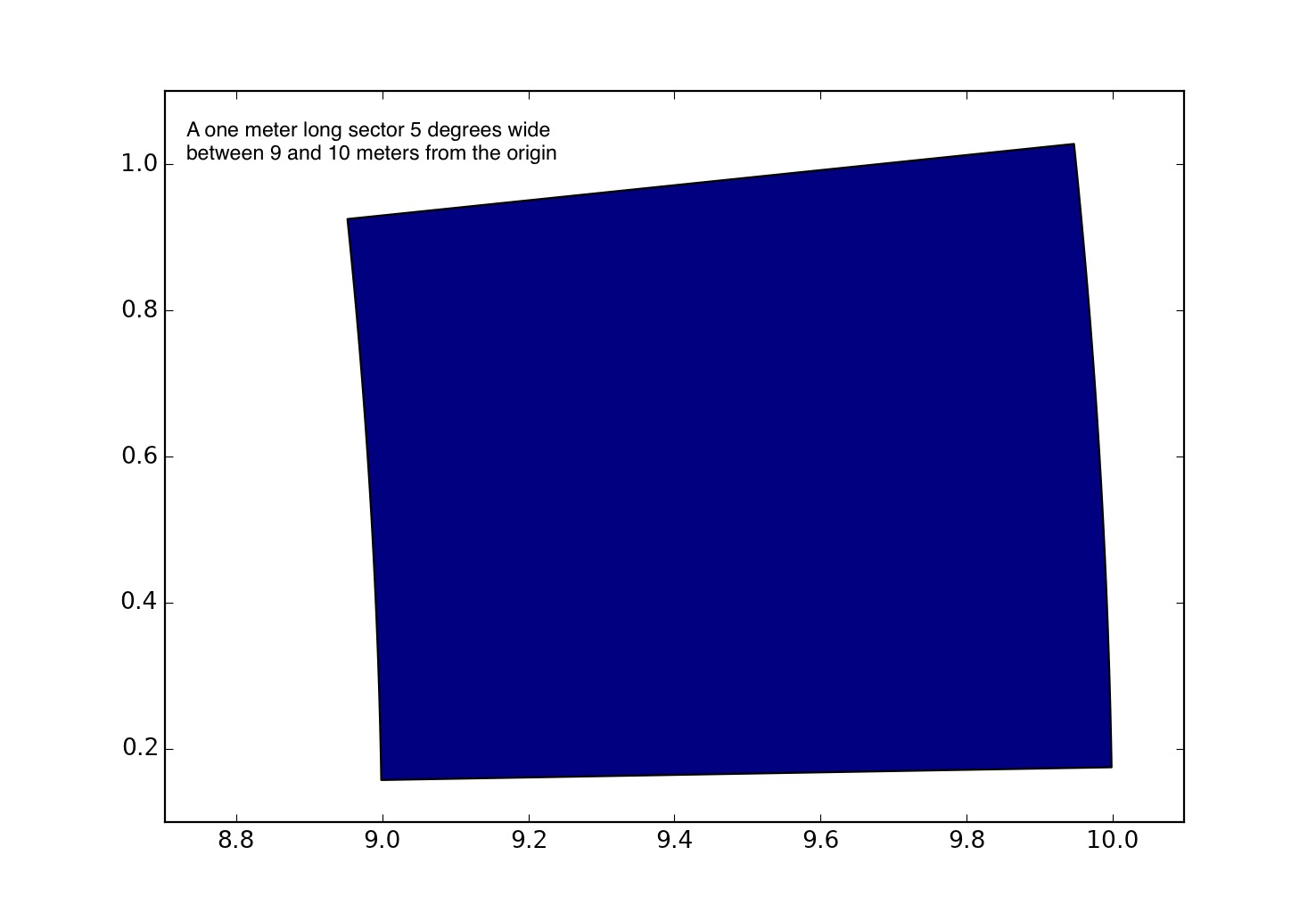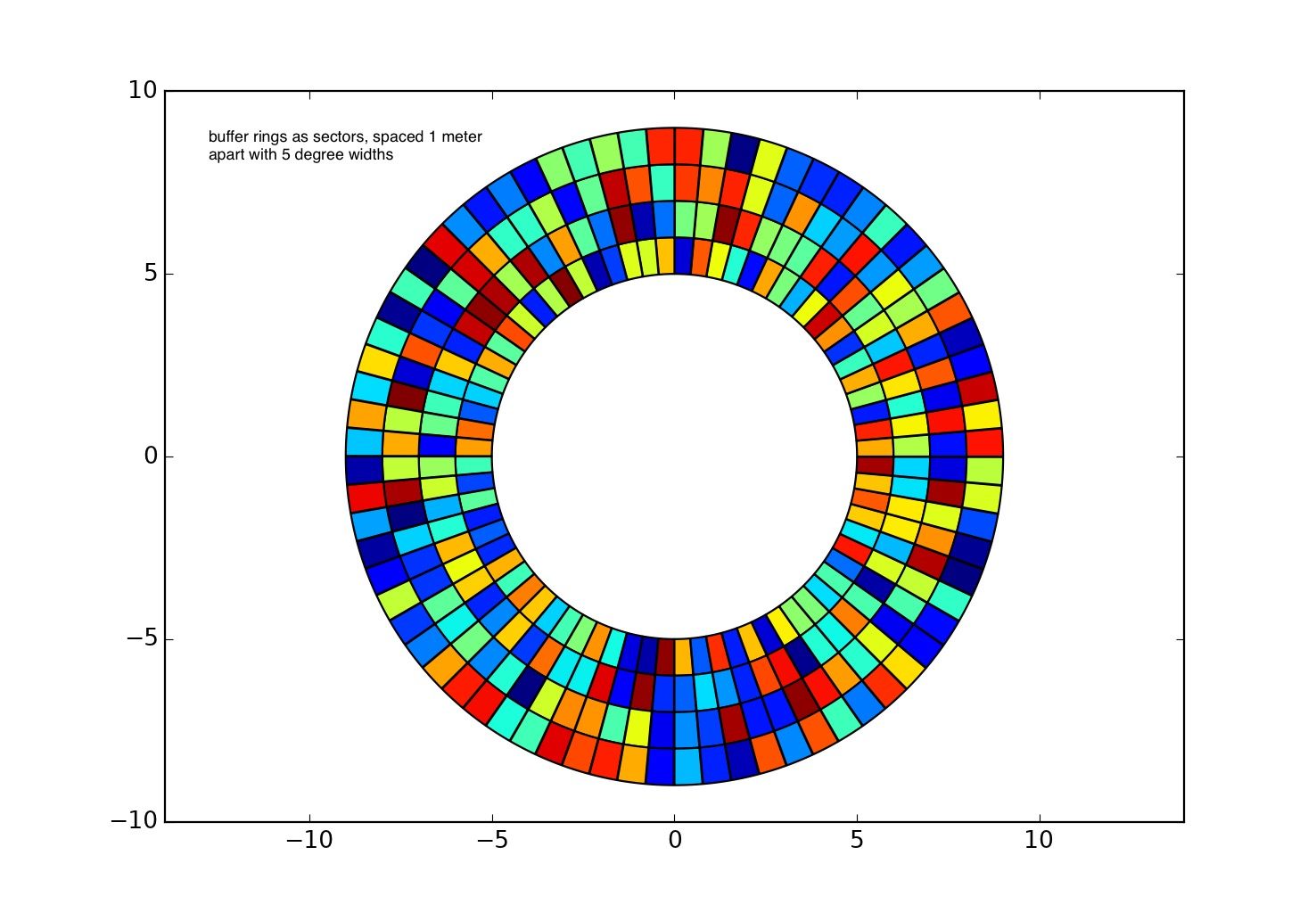With donut holes in the middle... the radiating line is a matplotlib artifact...I didn't want to waste time removing it.

Note that the ring widths are not equal... they need not be, you just set the threshold distances you want.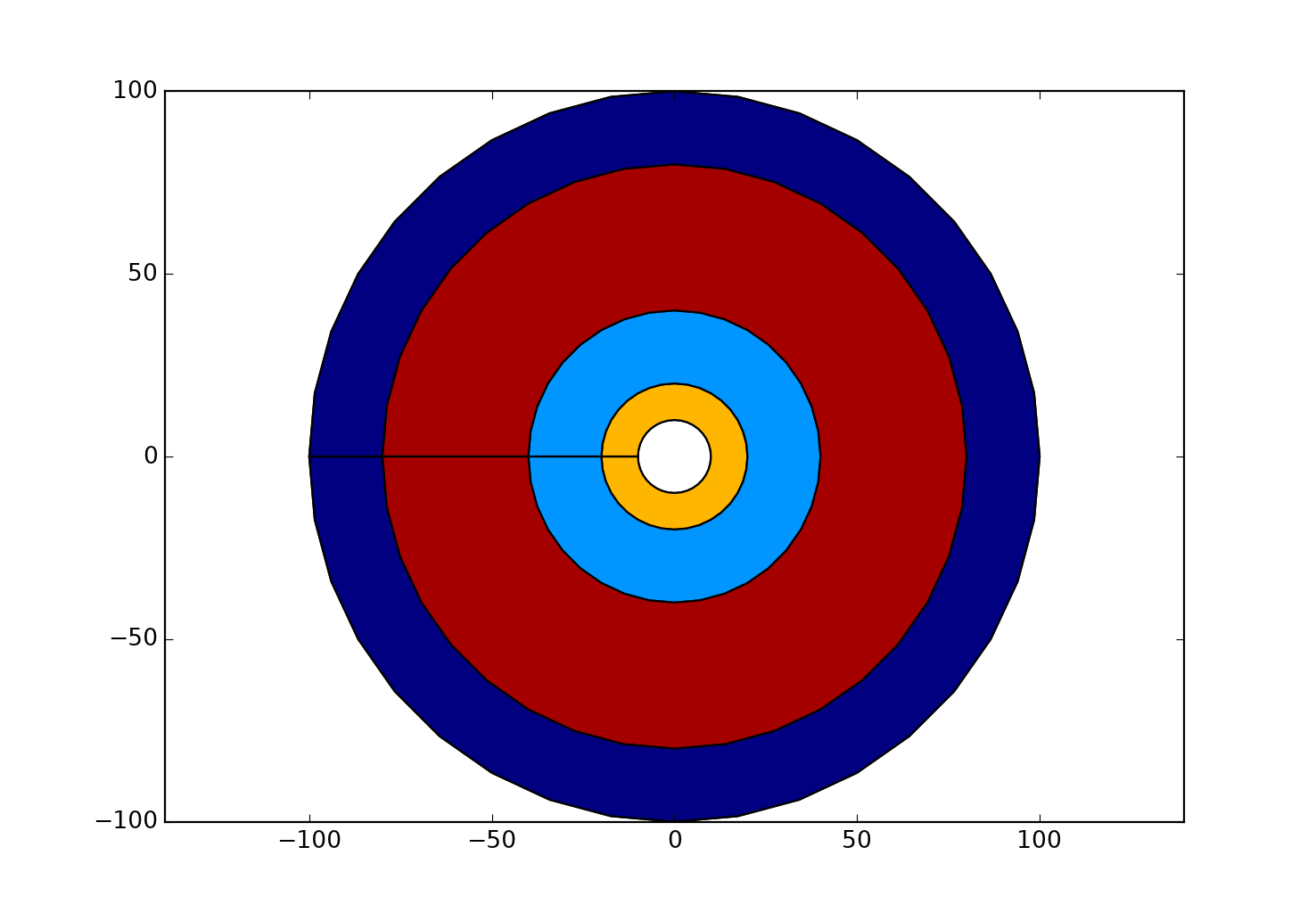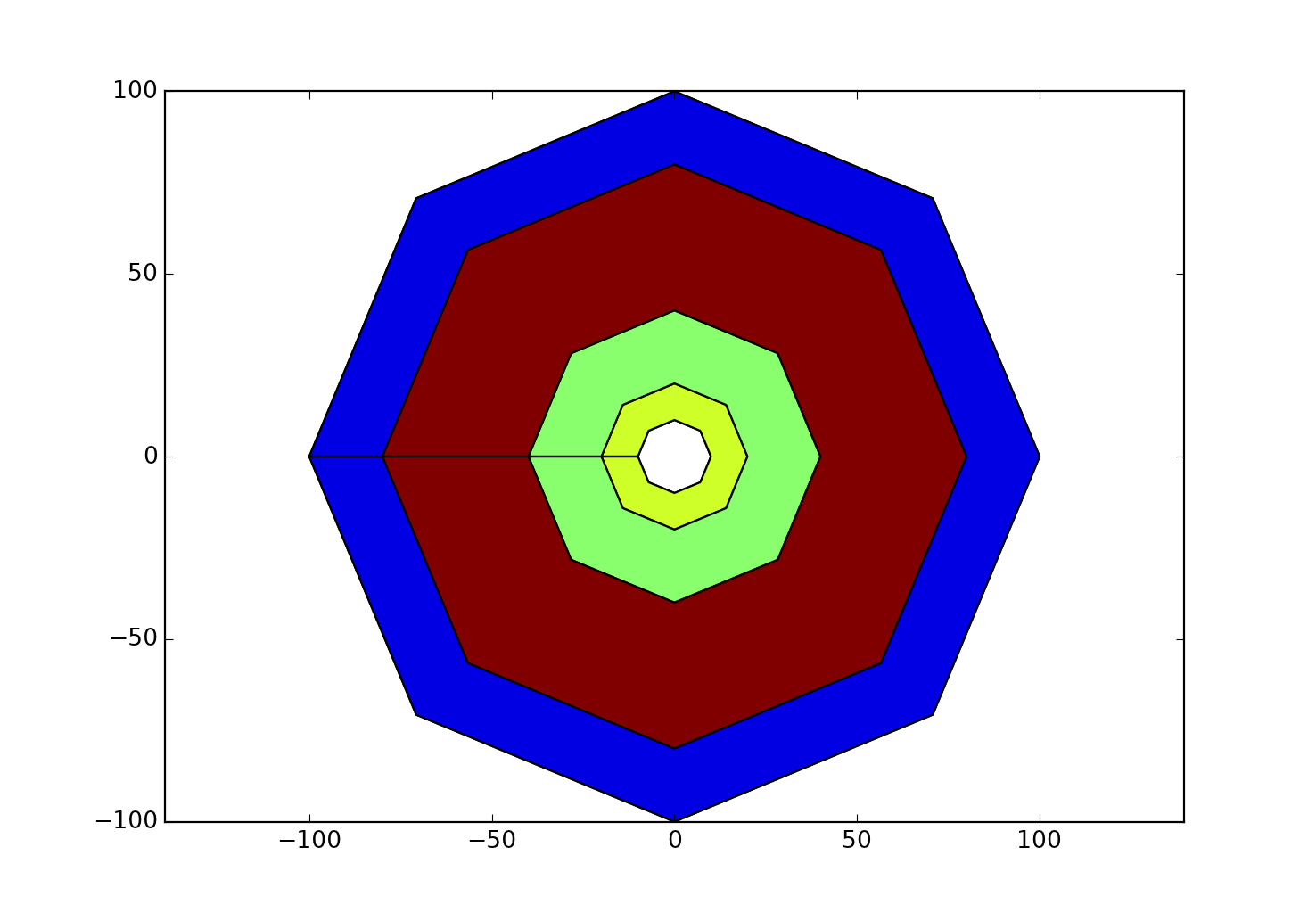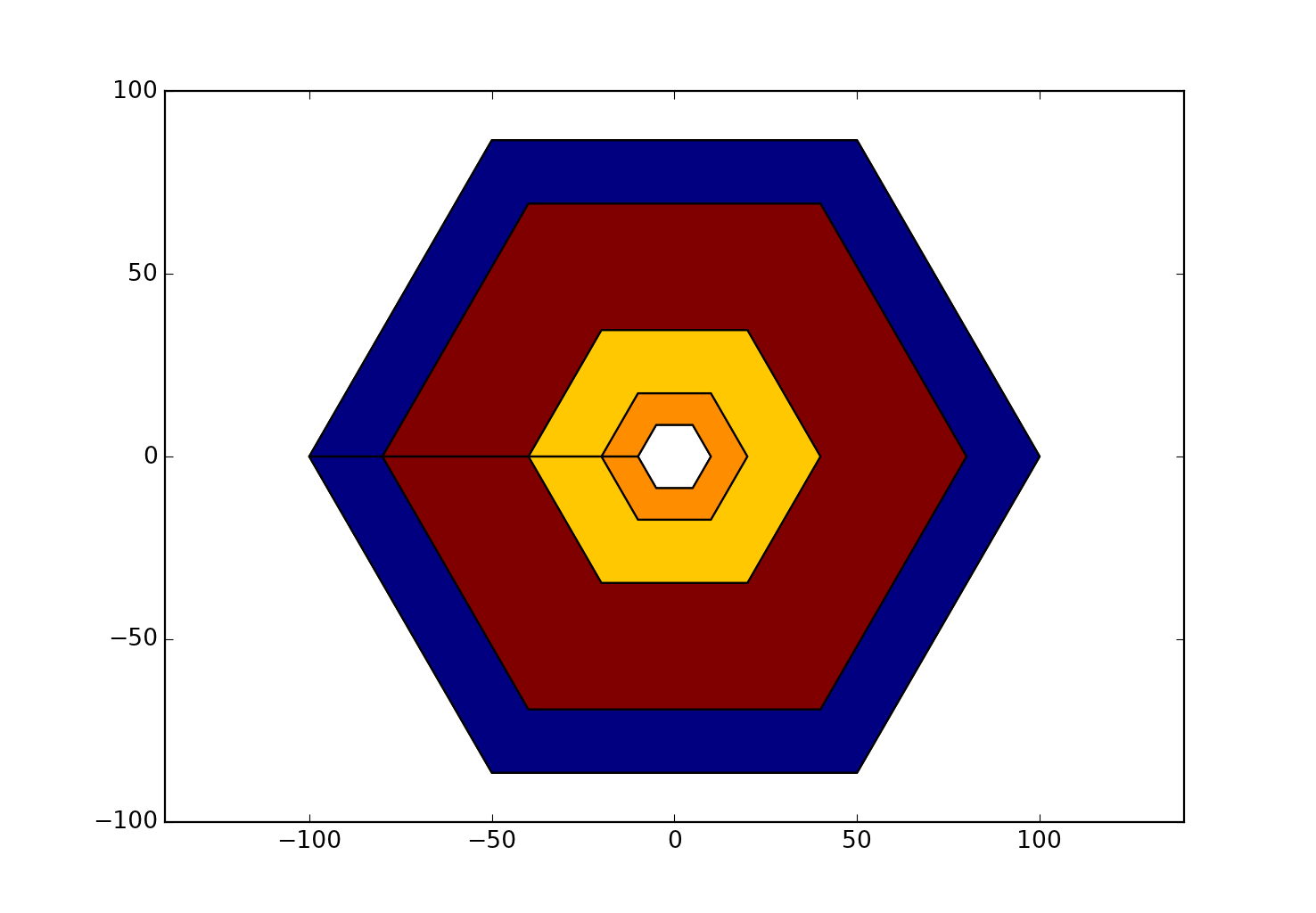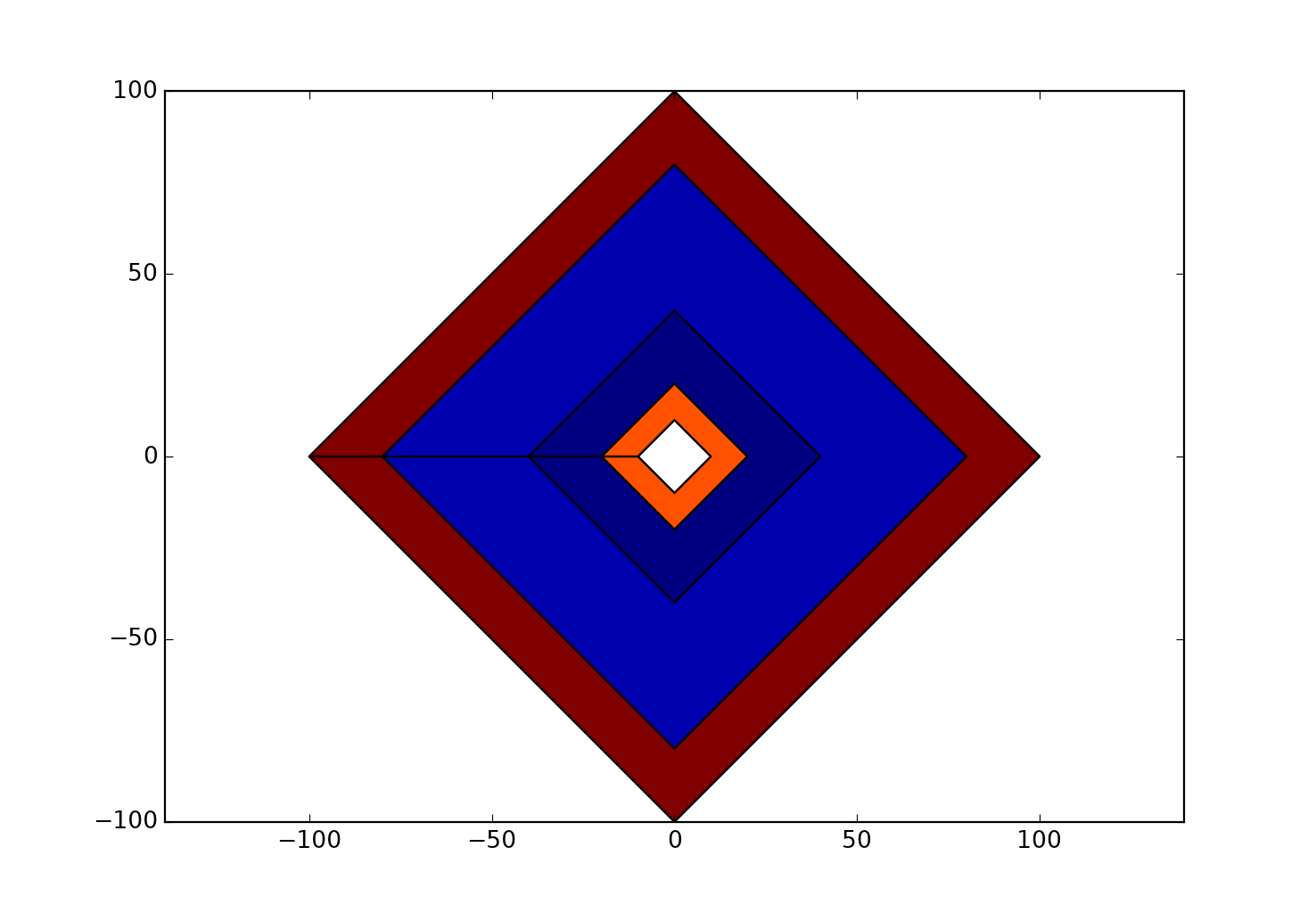These last two have a rotation set.  And the ellipse is the result of scaling the y-values and rotating the coordinates.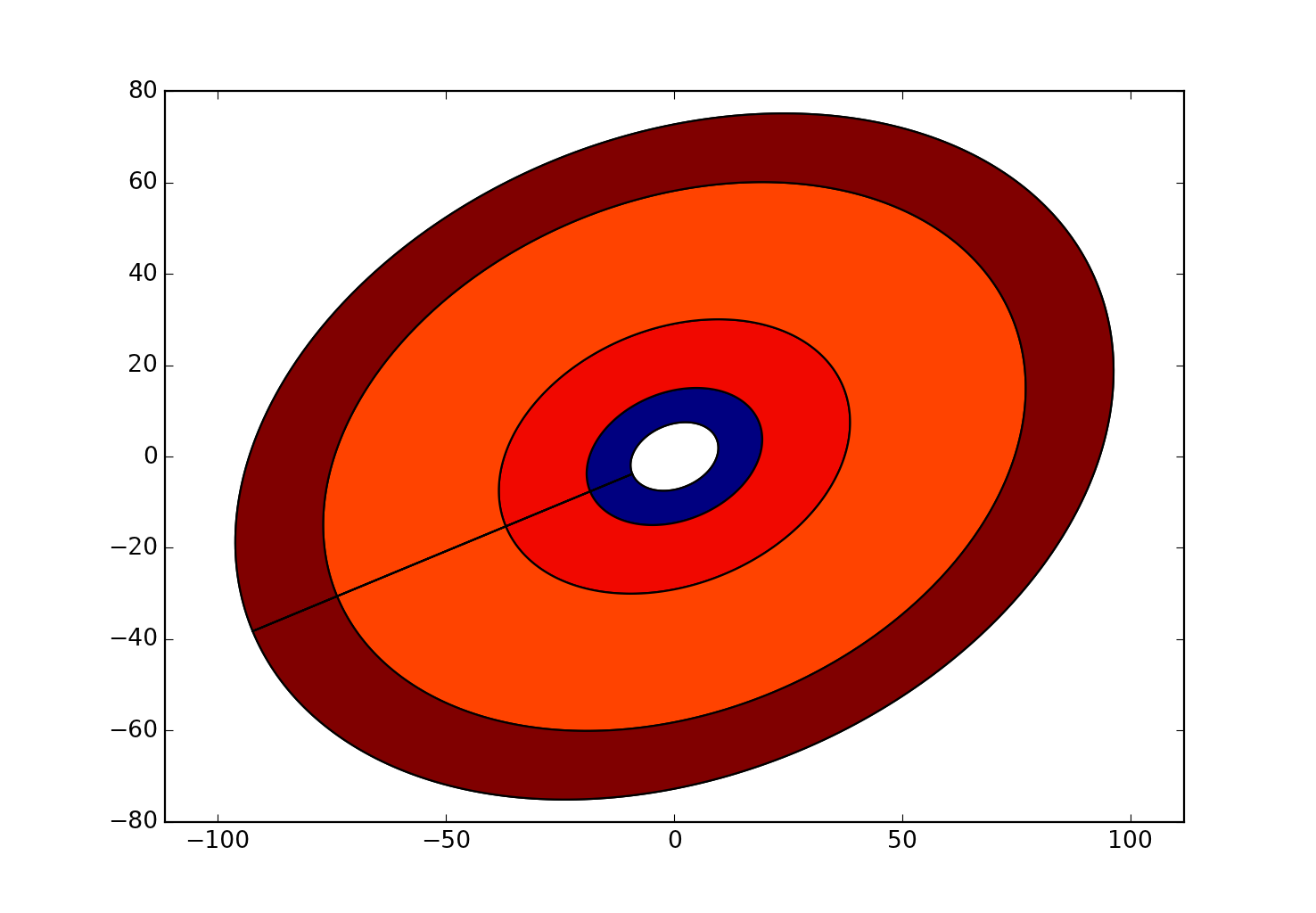As a simple example of the internal structure of the inputs, the following is an example of two triangles (3 points on a circle) with holes.  The data input is simply an input array as shown by the coordinates and the plotting routine handles the output.

``````a = buffer_ring(outer=10, inner=8, theta=120, xc=0.0, yc=0.0)
b = buffer_ring(outer=10, inner=8, theta=120, xc=10.0, yc=0.0)
a0 = Polygon(a, closed=False)
b0 = Polygon(b, closed=False)
#plot_([a,b])
a0p = a0.get_xy()
b0p = b0.get_xy()
props = b0.properties()‍‍‍‍‍‍‍‍‍‍‍‍‍‍‍‍‍‍‍‍‍‍‍‍‍‍‍‍‍‍‍‍‍‍‍‍‍‍‍‍‍‍‍‍‍‍‍‍‍‍‍‍‍‍‍‍‍‍‍‍‍‍‍‍‍‍‍‍‍‍‍‍``````

>>> a0p
array([[-10.000, 0.000],
[ 5.000, 8.660],
[ 5.000, -8.660],
[-10.000, -0.000],
[-8.000, -0.000],
[ 4.000, -6.928],
[ 4.000, 6.928],
[-8.000, 0.000]])
>>> b0p
array([[ 0.000, 0.000],
[ 15.000, 8.660],
[ 15.000, -8.660],
[ 0.000, -0.000],
[ 2.000, -0.000],
[ 14.000, -6.928],
[ 14.000, 6.928],
[ 2.000, 0.000]])

The triangles are as follows:The coordinates are above.

The outer rings go clockwise and the inner rings are counter clockwise.  You will notice that the first and last point of each ring are identical.